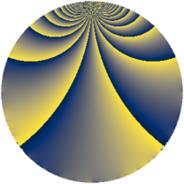# Properties

 Label 3549.2.kLevel $3549$ Weight $2$ Character orbit 3549.k Rep. character $\chi_{3549}(22,\cdot)$ Character field $\Q(\zeta_{3})$ Dimension $312$ Sturm bound $970$

# Related objects

## Defining parameters

 Level: $$N$$ $$=$$ $$3549 = 3 \cdot 7 \cdot 13^{2}$$ Weight: $$k$$ $$=$$ $$2$$ Character orbit: $$[\chi]$$ $$=$$ 3549.k (of order $$3$$ and degree $$2$$) Character conductor: $$\operatorname{cond}(\chi)$$ $$=$$ $$13$$ Character field: $$\Q(\zeta_{3})$$ Sturm bound: $$970$$

## Dimensions

The following table gives the dimensions of various subspaces of $$M_{2}(3549, [\chi])$$.

Total New Old
Modular forms 1028 312 716
Cusp forms 916 312 604
Eisenstein series 112 0 112

## Trace form

 $$312q - 4q^{2} - 160q^{4} - 16q^{5} + 24q^{8} - 156q^{9} + O(q^{10})$$ $$312q - 4q^{2} - 160q^{4} - 16q^{5} + 24q^{8} - 156q^{9} - 12q^{10} + 4q^{11} + 32q^{12} + 4q^{15} - 184q^{16} + 20q^{17} + 8q^{18} - 8q^{19} - 20q^{20} - 4q^{22} + 4q^{23} + 304q^{25} + 8q^{29} - 24q^{30} + 8q^{31} + 12q^{32} + 4q^{33} - 32q^{34} - 8q^{35} - 160q^{36} + 8q^{37} - 56q^{38} + 32q^{40} + 16q^{41} - 4q^{42} - 48q^{43} + 48q^{44} + 8q^{45} - 20q^{46} + 48q^{47} - 16q^{48} - 156q^{49} + 4q^{50} + 24q^{51} - 8q^{53} - 4q^{55} + 32q^{58} + 12q^{59} - 56q^{60} + 24q^{61} - 68q^{62} + 504q^{64} - 16q^{66} + 20q^{67} + 4q^{68} + 4q^{69} + 48q^{70} - 12q^{72} - 16q^{73} - 44q^{74} - 32q^{75} - 40q^{76} - 16q^{77} + 64q^{79} - 4q^{80} - 156q^{81} - 56q^{82} - 112q^{83} + 4q^{85} - 80q^{86} - 12q^{88} - 48q^{89} + 24q^{90} + 40q^{92} + 8q^{94} - 16q^{95} - 40q^{96} - 4q^{97} - 4q^{98} - 8q^{99} + O(q^{100})$$

## Decomposition of $$S_{2}^{\mathrm{new}}(3549, [\chi])$$ into newform subspaces

The newforms in this space have not yet been added to the LMFDB.

## Decomposition of $$S_{2}^{\mathrm{old}}(3549, [\chi])$$ into lower level spaces

$$S_{2}^{\mathrm{old}}(3549, [\chi]) \cong$$ $$S_{2}^{\mathrm{new}}(39, [\chi])$$$$^{\oplus 4}$$$$\oplus$$$$S_{2}^{\mathrm{new}}(91, [\chi])$$$$^{\oplus 4}$$$$\oplus$$$$S_{2}^{\mathrm{new}}(169, [\chi])$$$$^{\oplus 4}$$$$\oplus$$$$S_{2}^{\mathrm{new}}(273, [\chi])$$$$^{\oplus 2}$$$$\oplus$$$$S_{2}^{\mathrm{new}}(507, [\chi])$$$$^{\oplus 2}$$$$\oplus$$$$S_{2}^{\mathrm{new}}(1183, [\chi])$$$$^{\oplus 2}$$